# 前言

【关于Function(函数)翻译问题】

（PS：面向对象编程是在面向对象过程编程的基础上发展而来的）

# 函数概述

## 函数的概念

【实例】实现两数加法运算。

【代码示例】

#include <stdio.h>
int Plus();	//声明函数
int main(){
int x = 1;
int y = 2;
printf("%d", Plus(x, y));	//调用函数
return 0;
}
int Plus(int a,int b){	//函数定义
return a + b;
}


## 库函数

• 函数的功能
• 函数参数的数目和顺序，以及每个参数的意义以及类型
• 函数的返回值的意义以及类型
• 需要使用的包含文件

# 用户自定义函数

## 函数定义的格式

1. 无参数函数的定义格式

类型声明符 函数名称()
{
//函数体
}


类型声明符指定函数值的类型，即函数返回值的类型。如果一个函数没有返回值，该函数的返回值类型为void。函数名称的命名规则与变量的名称规则相同。

2. 有参数函数的定义格式

类型声明符 函数名称(形参列表声明){
//函数体
}


其中，类型声明符号指定函数返回值的类型，可以是任何有效类型，如果省略类型声明符号，系统默认函数的返回值为int类型。如果函数只是执行相关操作而不需要返回值，则可以使用void

有参函数在函数名称后的括号内必须有形式参数表，用于调用函数和被调用函数之间的数据传递，故必须对其进行类型声明，这由形式参数声明部分完成。一般情况下，函数执行需要多少原始外部数据，就有多少个形参数据，形参之间用逗号隔开。代码示例：

int Plus(int a,int b){	//定义自定义函数
return a + b;	//返回值
}

3. 空函数

C语言中可以有空函数，代码示例：

类型声明符 函数名()\
{
}


调用该函数，什么工作都不做。在主函数调用该函数，可以暂时表示某功能，后期扩充的时候填充该函数。

## 形式参数和实际参数

【实例】编写函数求三个整数中的最小值

【代码示例】

#include <stdio.h>
int MaxV(int a,int b,int c){
int max;
if (a>b)
{
max = a;
}else{
max = b;
}
if (max<c)
{
max = c;
}
return max;
}
int main(){
int x = 1;
int y = 2;
int z = 4;
printf("最大值为：%d", MaxV(x,y,z));
return 0;
}


【输出】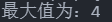【实例】求三个实数的平均值

【代码示例】

#include <stdio.h>
float Age(float a,float b,float c){
float x = (a + b + c) / 3;
return x;
}
int main(){
float x = 6;
float y = 3;
float z = 4;
printf("最大值为：%f", Age(x,y,z));
return 0;
}


【输出】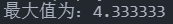## 函数的返回值

return 表达式;
return (表达式);
return;


1. return语句有双重作用：它使得函数从被调函数中退出，返回到调用的代码处，并向调用函数返回一个确定的值
2. 一个函数中可以用有多个return语句，执行到哪一个return语句，哪个return语句就起作用。

# 函数的调用

## 函数调用的一般形式

函数名(参数列表);


1. 如果调用无参函数，则实参列表可以没有，但是括号不能省略。
2. 实参列表的参数类型和个数必须与形参相同且顺序一致，多个实参之间用逗号隔开。

## 函数的调用方式

1. 把函数调用作为一个语句。此时一般不需要返回值，只需要执行特定的操作。

2. 在表达式中调用函数，这种表达式称为函数表达式。此时要求函数返回一个值参与运算，例如：

a=c*Plus(a,b,c);

3. 将函数调用作为另一个函数调用的参数。例如：

printf("和为：%d",Sum(a,b));


【实例】编写函数判断一个数是否是素数

【代码示例】

#include <stdio.h>
int IsPrimeint(int x){
if (x==1 || x==2)
{
return 0;
}
//如果一个数n可以被2~(n-1)之间的数除，则不是素数，返回1
for (int i = 0; i < x-2; i++)
{
if (x%(i+2)==0)
{
return 1;
break;
}
}
return 0;
}
int main(){
int prime;
scanf("%d", &prime);
if (IsPrimeint(prime)==1)	//根据返回值来判断是否是素数
{
printf("%d不是素数", prime);
}else{
printf("%d是素数", prime);
}
return 0;
}


【输出】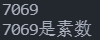## 函数的调用过程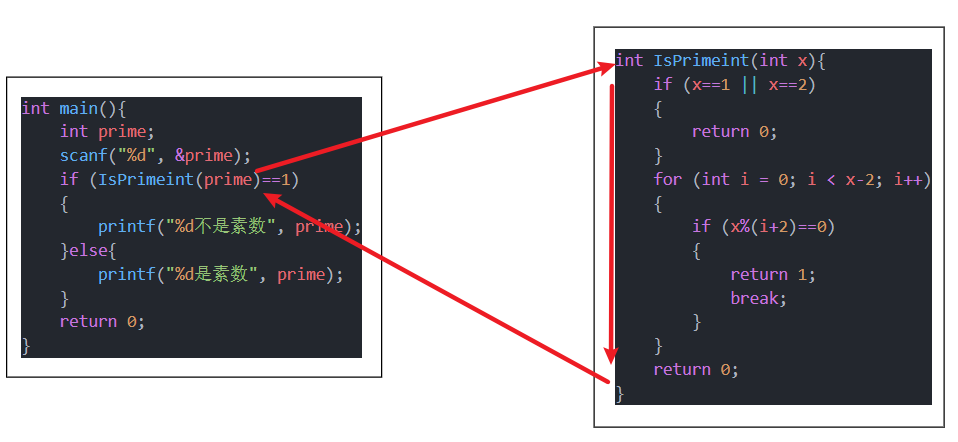## 函数的原型声明

1. 被调函数必须已经存在。

2. 如果使用库函数，需要提前引用相关库函数。

3. 如果使用用户自定义函数，并且该函数与主调函数在同一个文件中，这时被调用函数应该放在主调函数之前定义。如果函数调用的位置在函数定义之前，则在函数调用之前必须对所调用的函数进行函数原型声明，函数原型声明的语法格式如下：

类型声明符 函数名(形参表);


float average(float x,float y,float z);
//可以写为
float average(float,float,float);


## 函数的参数传递

1. 值传递

在函数调用时，实参将其值传递给形参。这种传递方式即为值传递。

C语言规定，实参对形参的数据传递是值传递，即单向传递，也就是只能由实参传递给形参而不能由形参传递回来给实参。这是因为，在内存中，实参和形参占用不同的存储单元。在调用函数时，给形参分配存储单元，并将实参对应的值传递给形参，调用结束后，形参的存储单元会被释放，实参的存储单元仍要保留维持原值。因此，在执行一个被调用函数时，形参的变化不会改变实参的值。

2. 地址传递

地址传递指的是函数调用时，实参将某些量的地址传递给形参。这样实参和形参指向同一个内存空间，在执行被调函数的过程中，对形参所指向的空间中的内容改变能够直接影响到实参的值。

在地址传递方式下，形参和实参可以是指针变量（欠-指针）

# 函数的嵌套调用和递归调用

## 函数的嵌套调用

C语言中的函数的定义是相互平行的，在定义函数时，一个函数不能包含另一个函数。但是，一个函数在被调用的过程中可以调用其他函数，这就是函数的嵌套调用。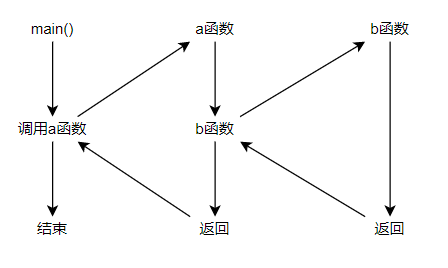【实例】计算1+$2!$+$3!$+·····+$10!$。（使用嵌套函数）

【代码示例】

#include <stdio.h>
int Sum(int);	//自定义函数原型声明
int Fac(int);	//自定义函数原型声明
int sum = 0;	//和的值
int main(){
for (int i = 0; i < 10; i++)
{
Sum(Fac(i + 1));
}
printf("阶乘的和为：%d", sum);
sum = 0;
return 0;
}
//求和
int Sum(int num){
sum += num;
return sum;
}
//求阶乘
int Fac(int num){
int res = 1;
for (int i = 0; i < num; i++)
{
res *= (i + 1);
}
return res;
}


【输出】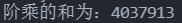## 函数的递归调用

【实例】利用递归安抚计算$n!$。

【代码示例】

#include <stdio.h>
int facRes = 1;	//阶乘结果返回值
int Fac(int);	//自定义阶乘函数原型声明
int main(){
int num = 1;
scanf("%d", &num);	//获取要求的阶乘
printf("%d!阶乘的结果为：%d",num, Fac(num));
return 0;
}
//阶乘计算
int Fac(int num){
if (num!=1)
{
facRes *= num;
Fac(num - 1);	//递归调用
}
return facRes;
}


【输出】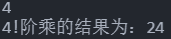# 数组作为函数的参数

## 一维数组作为函数参数

【实例】求一组整数的平均值（一维数组）

【代码示例】

#include <stdio.h>
float Avg(int x[]);
int main(){
int n = {1, 2, 3, 4, 5};
printf("平均值为：%f", Avg(n));
return 0;
}
//求平均函数
float Avg(int x[]){
int sum = 0;
for (int i = 0; i < 5; i++)
{
sum += x[i];
}
return sum / 5;
}


【输出】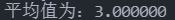## 二维数组作为函数的参数

void find(char x);
//或者
void find(char x[]);


void find(char x[][]);


//实参为：
int array;
//形参可以定义为：
void Test(int array);
//形参或者为：
void Test(int array);


【实例】实现两个 3 $\times$ 4 矩阵A和B的加法运算。

【代码示例】

#include <stdio.h>
void Plus(int a[], int b[]);	//自定义函数原型声明
int res;	//矩阵和结果
int main(){
int a;
int b;
for (int i = 0; i < 3; i++)	//获取需要计算的矩阵A
{
printf("请输入矩阵A第%d行数据 | ",i+1);
scanf("%d %d %d %d", &a[i], &a[i], &a[i], &a[i]);
}
printf("--------------\n");
for (int i = 0; i < 3; i++)	//获取需要计算的矩阵B
{
printf("请输入矩阵B第%d行数据 | ",i+1);
scanf("%d %d %d %d", &b[i], &b[i], &b[i], &b[i]);
}
printf("矩阵的和为:\n");
Plus(a, b);	//调用自定义函数
return 0;
}
//矩阵和计算函数
void Plus(int a,int b){
for (int i = 0; i < 3; i++)
{
for (int j = 0; j < 4; j++)
{
res[i][j] = a[i][j] + b[i][j];
}
printf("%d %d %d %d\n", res[i], res[i], res[i], res[i]);
}
}


【输出】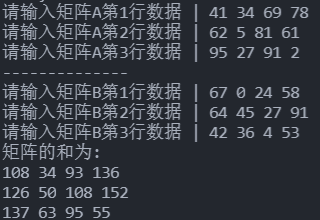# 局部变量和全局变量

C语言程序是由一些函数组成的。每个函数都是相对独立的代码块，这些代码只局限于该函数。因此，在非特殊说明下，一个函数的代码对于程序的其他部分来说是隐藏的，它既不会影响程序的其他部分，也不会受程序其他部分的影响。也就是说一个函数的代码和数据不可能与另一个函数的代码和数据相互作用。这是因为它们分别有着自己的作用域。根据作用域的不同，变量分为两种类型：局部变量和全局变量

## 局部变量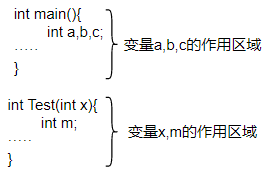1. 主函数main()中定义的变量也是局部变量，仅在main()函数中有效。
2. 形参也是局部变量，只能在定义它的函数中有效。
3. 不同的函数中，可以使用相同名称的局部变量，它们代表不同的对象，互不干扰。

【实例】判断下述代码的运行结果

【代码示例】

#include <stdio.h>
int main(){
int x = 10;
{
int x = 20;
printf("%d ", x);
}
printf("%d", x);
return 0;
}


【输出】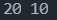## 全局变量

【实例】extern关键字使用

【代码示例】

#include <stdio.h>
int main(){
extern int a, b;	//全局变量原型声明
a = 1;
b = 2;
printf("a=%d b=%d", a,b);
return 0;
}
int a, b;


【输出】 a=1 b=2

【实例】全局变量与局部变量同名，分析结果

【代码示例】

#include <stdio.h>
int a = 10;
int main(){
void Test(int);
int a = 1;
Test(a);
printf("主函数变量%d", a);
return 0;
}
void Test(int b){
printf("%d\n", b+ ++a);
}


【输出】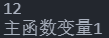1. 全局变量使函数的执行依赖于外部变量，降低了程序的通用性。模块化程序设计要求各个模块之间的“关联性”应尽量的小，函数尽可能是封闭的，只通过参数与外界发生联系。
2. 降低程序的清晰性。
3. 全局变量在整个程序的执行过程中都会占用存储空间。

# 变量的存储类别

• 程序区：存放用户程序
• 静态存储区：存放全局变量，静态局部变量和外部变量
• 动态存储区：存放局部变量，函数形参变量

CPU寄存器存放寄存器变量

【关于静态局部变量的作用】

• 变量如果没有赋初值，对于一般的变量其值系统给的是NULL，静态局部变量系统会赋予初始值0；

• 静态局部变量占用内存，其值会一直保存，可以适用于需要多次调用某方法，但是值要增加的条件等，代码示例：

#include <stdio.h>
int main(){
int Plus();
for (int i = 0; i < 3; i++)
{
printf("主函数变量%d", Plus());	//调用三次，获得三次不同的结果
}
return 0;
}
int Plus(){
static int a;	//静态局部变量
a++;	//自增
return a;	//返回结果
}


输出结果：主函数变量1 主函数变量2 主函数变量3

C语言有4种变量存储类别声明符，用来通知编译程序采用哪种方式存储变量，这4种变量存储类别声明符是：

• 自动变量声明符auto（一般省略）
• 静态变量声明符static
• 外部变量声明符extern
• 寄存器变量声明符register

## 局部变量的存储类型

### 自动变量

[auto] 类型声明符 变量名;


auto int a;
//等价于
int a;


### 局部静态变量

static 类型声明符 变量名;


1. 局部静态变量是在静态存储区分配存储单元的。一个变量被声明为静态，在编译时即分配存储空间，在整个程序运行期间都不释放。因此，函数调用结束后，它的值并不消失，其值能够保持连续性
2. 局部静态变量是在编译过程中赋予初值的，且只赋予一次初值，在程序运行时其初值已定，以后每次调用函数时，都不再赋予初值，而是保留上一次函数调用结束时的结果。
3. 局部静态变量在未显式初始化时，编译系统把它们初始化为 0（整型变量），0.0（实型变量），\0(空字符)（字符型变量）

### 寄存器变量

【实例】寄存器变量的应用

【代码示例】

#include <stdio.h>
int main() {
register int i, j;
for (i = 1; i < 10; i++)
{
for (j = 1; j < i+1; j++)
{
printf("%d * %d = %d ", j, i, j * i);
}
printf("\n");
}
return 0;
}


【输出】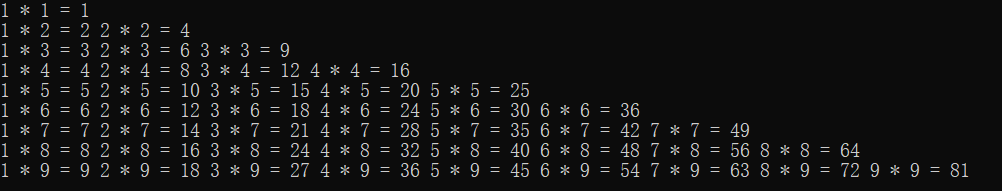1. 只有局部自动变量和形参可以作为寄存器变量，其他（如全局变量，局部静态变量则不行）
2. 只有int,char和指针类型变量可以定义为寄存器类型，而long,doublefloat型变量不能设定为寄存器类型，因为它们的数据长度已经超出通用寄存器本身的位长。
3. 可用于变量空间分配的寄存器的个数依赖于具体的机器

## 全局变量的存储类别

### 外部全局变量

【实例】调用其他文件的全局变量

【代码示例】

//文件一代码
#include <stdio.h>
void Test();	//在文件二自定义函数原型声明
int a = 32;	//全局变量声明定义
int main() {
Test();	//调用文件二自定义函数
return 0;
}
//文件二代码
#include <stdio.h>
extern int a;	//文件一全局变量原型声明
void Test() {
int c = a;
printf("%d", c);
}


【输出】32

1. extern只能用来声明变量，不能用来定义变量。因为它不会生成新的变量，只是表示该变量已在其他地方有过定义。

2. extern用来声明变量时，类型名可以写，也可以不写，例如：

extern int a;
//或者
extern a;

3. extern不能用来初始化变量，例如：

extern int a=1;


### 静态全局变量

static int a;


# 内部函数和外部函数

C语言由函数组成的，这些函数既可以在一个文件中也可以在多个不同的文件中，根据函数的使用范围，可以将其分为内部函数和外部函数

## 内部函数

static 类型声明符 函数名(形参表);


## 外部函数

extern 类型声明符 函数名(形参表);


Q.E.D.

••